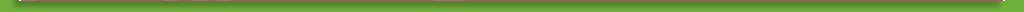# The Ping-Pong Ball Paradox

The Ross-Littlewood Paradox questions if there’s infinity or nothing. Find out how.

Counting is pretty easy, right? It’s one of the first things we ever learn about math. Did you know there is a way to count where you can get two completely different answers? It’s called the Ross-Littlewood paradox or sometimes the Ping-Pong ball problem. Stick around to find out exactly how you can count the same things and get different answers.

The basic problem with the Ross-Littlewood paradox is you have an infinite number of Ping-Pong balls. You also have a vase that can hold an infinite number of Ping-Pong balls. The next is that you add and subtract Ping-Pong balls at set time intervals. That is where things get complicated.

Infinity Ping-Pong ballsIf you want an infinity number of balls in the vase you have to count a specific way. First, all the balls need to be numbered sequentially, 1, 2, 3, 4… At one minute until noon you add balls one through ten, but then remove ball ten. At 30 seconds until noon, you add balls 11–20 and remove ball 20. At 15 seconds until noon, you add 21–30 and then remove ball 30.

So at every step, you divide the time until noon in half and add the next round of balls and remove one. So how many are in the vase at noon? Every step adds ten balls and removes one, a net gain of nine. To know how many balls are in the vase at noon we need to know how many times we repeated the process. Simple right? Not exactly. Every step takes place in half the time as the last. Theoretically, time is infinitely divisible, so technically there is always more time to add more balls. Thus you end up with an infinite number of balls.

But do you always?

An empty vase

Instead of taking out the tenth ball at every step, what if you take out balls in sequential order? Ball one at the first step, ball two on the second, ball three on the third, and so on? You’re still taking out one ball for every nine you put in. But at noon, the vase is empty. How?

Remember how there are an infinite number of steps? Well, if you take out a ball one at step one and there are infinite steps, then you take out infinite balls. Leading to no balls in the vase.

The difference seems inconsequential. You add in ten balls each time. You remove one ball each time. Leaving behind nine at each step. It’s how you count that matters.

Correspondence

Normally when we think of counting, we think of a one-to-one correspondence. That means we count one per every action. That’s why the empty vase version works. For every step, the ball with that number is removed. The counting of steps and the counting of Ping-Pong balls is a one-to-one correspondence.

In the case where you remove every tenth ball, you don’t create a one-to-one correspondence; the balls don’t have a matching step.

Conclusion

It’s not truly a paradox. There is no error in the mathematical logic. It simply isn’t the arithmetic that we are used to. That is the thing with math: it is a very complex thing. Just because a problem doesn’t make sense in one view, math probably has another way of looking at it where it does make sense. Too bad the Ross-Littlewood paradox doesn’t work on your allowance.

# Poll

You get to plant a magical garden! What do you plant?